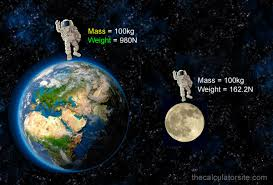## 4ESO Física i Química : ARTICLE 128 : WEIGHT AND MASS DIFFERENCE. WEIGHT ON OTHER PLANETS.

Publicado: 03/05/2017 08:45 por easyfisquim en 4ESO FiQ 2016-2017 ENGLISH (from article 47).- Now read a few definitions about the difference between mass and weight. After reading them try to write your own definition with your own words about the difference between mass and weight :

Definition 1 :

In common usage, the mass of an object is often referred to as its weight, though these are in fact different concepts and quantities. In scientific contexts, mass refers loosely to the amount of "matter" in an object (though "matter" may be difficult to define), whereas weight refers to the force exerted on an object by gravity. In other words, an object with a mass of 1.0 kilogram will weigh approximately 9.81 newtons on the surface of the Earth (its mass multiplied by the gravitational field strength).

(The newton is a unit of force, while the kilogram is a unit of mass.)

Its weight will be less on Mars (where gravity is weaker), more on Saturn, and negligible in space when far from any significant source of gravity, but it will always have the same mass.

Definition 2 :

A better scientific definition of mass is its description as being composed of inertia, which is the resistance of an object being accelerated when acted on by an external force. Gravitational "weight" is the force created when a mass is acted upon by a gravitational field and the object is not allowed to free-fall, but is supported or retarded by a mechanical force, such as the surface of a planet. Such a force constitutes weight. This force can be added to by any other kind of force.

Definition 3 :

While the weight of an object varies in proportion to the strength of the gravitational field, its mass is constant (ignoring relativistic effects) as long as no energy or matter is added to the object. Accordingly, for an astronaut on a spacewalk in orbit (a free-fall), no effort is required to hold a communications satellite in front of him; it is "weightless". However, since objects in orbit retain their mass and inertia, an astronaut must exert ten times as much force to accelerate a 10‑ton satellite at the same rate as one with a mass of only 1 ton.

On Earth, a swing seat can demonstrate this relationship between force, mass, and acceleration. If one were to stand behind a large adult sitting stationary on a swing and give him a strong push, the adult would temporarily accelerate to a quite low speed, and then swing only a short distance before beginning to swing in the opposite direction. Applying the same impetus to a small child would produce a much greater speed.

Definition 4 :

Definition 5 :

* Have you really understood the difference ?  then...try to do these exercises :

Wording  1:

You are on the earth and have a bag of apples which weights 85N.  If you were to carry
the bag of apples to the surface of the moon, where the gravitational field is
only 1.67 N/kg, how much would the apples weigh?

Wording 2 :

An 80 kg person on
top of Mt. Everest would weigh only 781.6 N.
How strong is the Earths gravitational field on top of Mt. Everest?

Wording 3 :

If you went to the
moon, how much would you weigh?

Comentarios  Ir a formulario